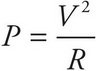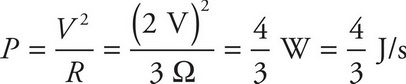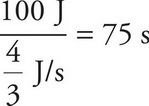# AP Physics 1 Question 416: Answer and Explanation

### Test Information

Question: 416

4. If the voltage drop across a 3 Ω resistor is 2 V, how long will it take for the resistor to dissipate 100 J of energy?

• A. 75 s
• B. 100 s
• C. 125 s
• D. 150 s

The equationgivesThe amount of time it takes for the resistor to dissipate 100 J of energy is equal to.